Latest Banking jobs   »

# Quantitative Aptitude Quiz For LIC AAO 2023- 18th January

Directions (1-5): In each question two equations numbered (I) and (II) are given. You have to solve both the equations and mark appropriate answer.
(a) If x<y
(b) If x>y
(c) If x≥y
(d) If x≤y
(e) If x=y or no relation can be established.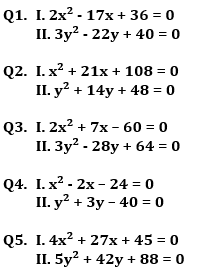(a) x > y
(b) x ≥ y
(c) x < y
(d) x ≤ y
(e) x = y or no relation can be established between x and y.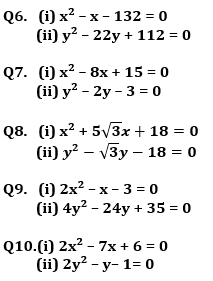Directions (11-15): In each question two equations numbered (I) and (II) are given. You should solve both the equations and mark appropriate answer.
(a) If x=y or no relation can be established
(b) If x>y
(c) If x<y
(d) If x≥y
(e) If x≤y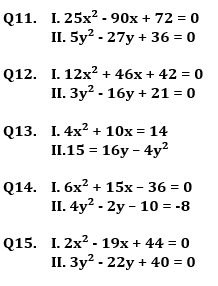Solutions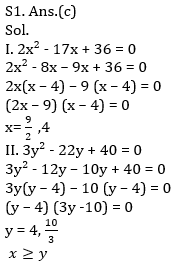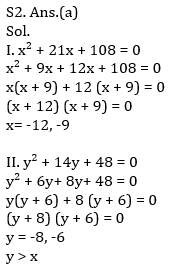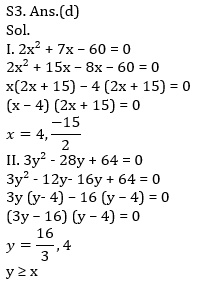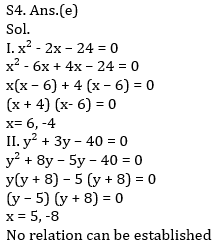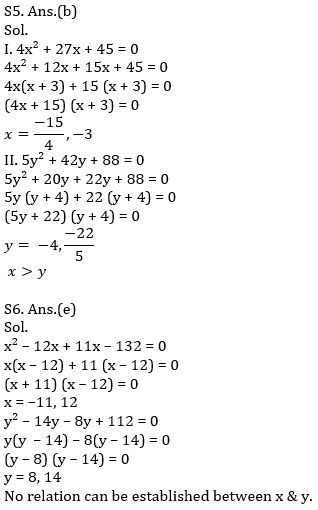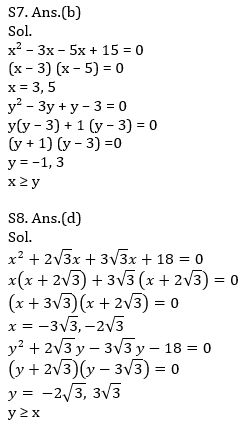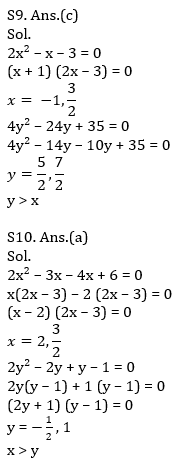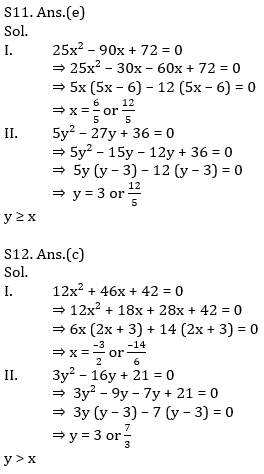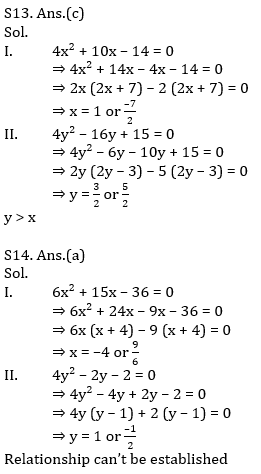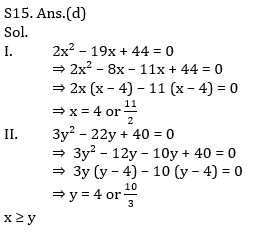## FAQs

### When will the LIC AAO Prelims exam be held?

The LIC AAO Prelims exam will be held on 17th & 20th of February 2023 (tentatively).

#### Congratulations!